Homework Help Question & Answers

Your Answer:

#### Post as a guest

Your Name:

What's your source?

#### Earn Coin

Coins can be redeemed for fabulous gifts.

Not the answer you're looking for? Ask your own homework help question. Our experts will answer your question WITHIN MINUTES for Free.
Similar Homework Help Questions
• ### uestion #1:Solving for the Goods MarketSuppose an economy can be described by the following set of...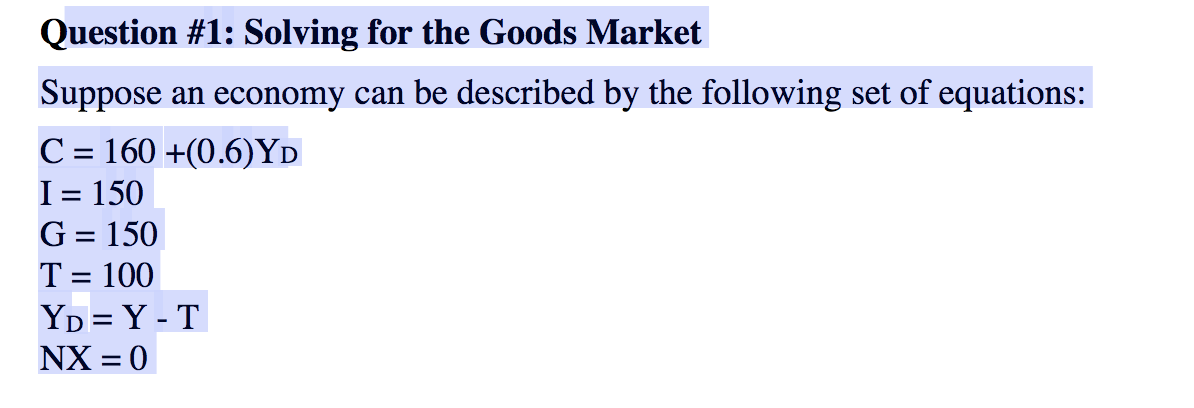uestion #1:Solving for the Goods MarketSuppose an economy can be described by the following set of equations:C = 160+(0.6)YDI = 150G = 150T = 100YD= Y -TNX = 0 (g) What is the marginal propensity to consume (mpc)?(h) What is the marginal propensity to save (mps)?(i) Calculate the multiplier. [Hint: The multiplier = 1/(1-mpc)](j) Calculate the level of autonomous spending. [Hint: Autonomous spending = C0+ I + G –(mpc x T)] Question #1: Solving for the Goods Market Suppose an...

• ### 1) Suppose an economy is characterized by the following equations. Y = C+/+G Y = 10,500...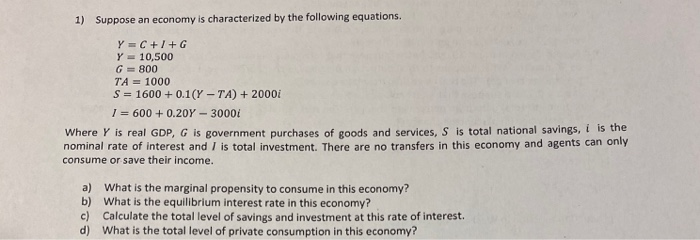1) Suppose an economy is characterized by the following equations. Y = C+/+G Y = 10,500 G = 800 TA = 1000 S = 1600+ 0.1(Y-TA) + 20001 1 = 600+ 0.20Y - 30001 Where Y is real GDP, G is government purchases of goods and services, S is total national savings, is the nominal rate of interest and I is total investment. There are no transfers in this economy and agents can only consume or save their income. a)...

• ### Econ 105A Jang-Ting Guo Handout/Homeowork 4 1. The following equations describe an economy. Think of C,...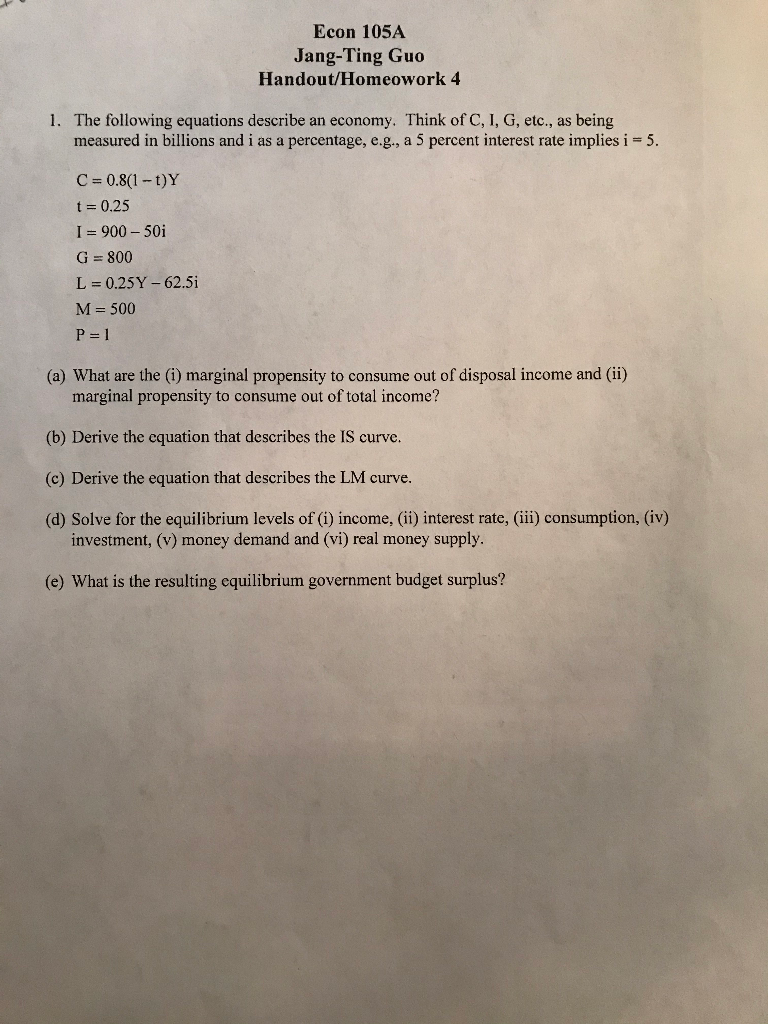Econ 105A Jang-Ting Guo Handout/Homeowork 4 1. The following equations describe an economy. Think of C, 1, G, etc., as being measured in billions and i as a percentage, e.g., a 5 percent interest rate implies i = 5. C = 0.8(1-t)Y t = 0.25 I = 900 - 50i G = 800 L = 0.25Y - 62.5i M=500 P=1 (a) What are the (1) marginal propensity to consume out of disposal income and (ii) marginal propensity to consume out...

• ### . YOU ARE GIVEN THE FOLLOWING INFORMATION ABOUT THE ECONOMY OF ENGLAND. PLEASE ANSWER QUESTIONS A...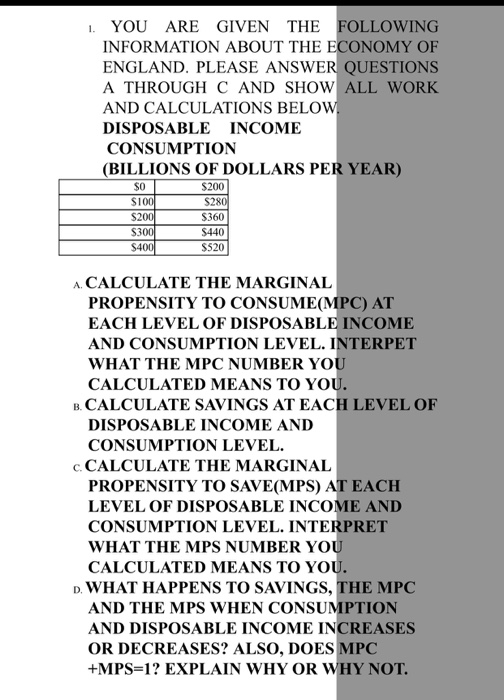. YOU ARE GIVEN THE FOLLOWING INFORMATION ABOUT THE ECONOMY OF ENGLAND. PLEASE ANSWER QUESTIONS A THROUGH C AND SHOW ALL WORK AND CALCULATIONS BELOVW DISPOSABLE INCOME CONSUMPTION (BILLIONS OF DOLLARS PER YEAR) $200$100 S20 S30 $40 S360$440 S520 A CALCULATE THE MARGINAL PROPENSITY TO CONSUME(MPC) AT EACH LEVEL OF DISPOSABLE INCOME AND CONSUMPTION LEVEL. INTERPET WHAT THE MPC NUMBER YO CALCULATED MEANS TO YO B. CALCULATE SAVINGS AT EACH LEVEL OF DISPOSABLE INCOME AND CONSUMPTION LEVEL. c....

• ### Need help on this question, I tried the ones i know

Need help on this question, I tried the ones i know.a The consumption function is C = 1.5 + 0.75(Y-T). What is the marginal propensity to consume,MPC? What is the marginal propensity to save, MPS?ans: MPC=0.75 and MPS=1-MPC=0.25 correct?b The trade balance is TB = 5(1-[1/E])-0.25(Y-8).What is the marginal propensity to consume foreign goods,MPCF ? What is the marginal propensity to consume home goods, MPCH?c The investment function is I=2-10i. What is investment when the interest rate i is equal...

• ### QUESTION TWO An economy is represented by the following set of equations: Y = C +...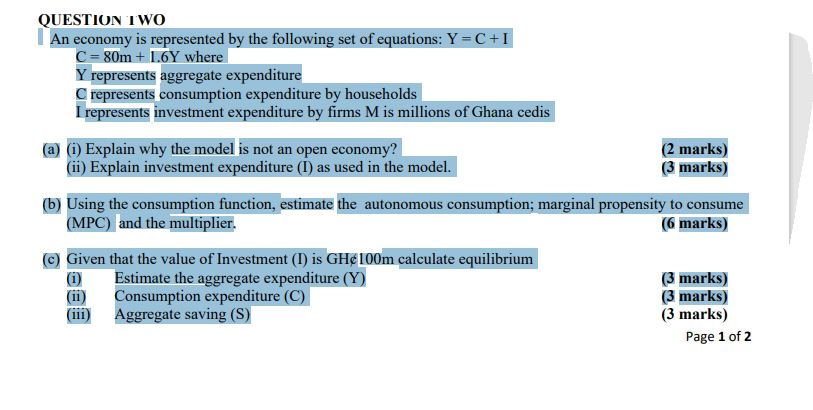QUESTION TWO An economy is represented by the following set of equations: Y = C + I C = 80m + 1.6Y where Y represents aggregate expenditure C represents consumption expenditure by households I represents investment expenditure by firms M is millions of Ghana cedis (a) (i) Explain why the model is not an open economy? (2 marks) (ii) Explain investment expenditure (I) as used in the model. (3 marks) (b) Using the consumption function, estimate the autonomous consumption; marginal...

• ### 1. Consider the following consumption function and the national income identity. C=0.01Y2 +0.8Y+200 Y=C+S Where, C...

1. Consider the following consumption function and the national income identity. C=0.01Y2 +0.8Y+200 Y=C+S Where, C is consumption and Y is national income, and S is saving a) Calculate the value of marginal propensity to consume (MPC) when Y=8 b) Find the expression for savings function and using that function calculate marginal propensity to save (MPS) when Y= 8.

• ### For an economy described by the following equations: C = 1,800 + 0.6 (Y – T)...

For an economy described by the following equations: C = 1,800 + 0.6 (Y – T) I p = 900 G = 1,500 NX = 100 T = 1,500 Y* = 9,000 Assume that the multiplier for this economy is 2.5. Find the effect on short-run equilibrium output of: a. An increase in government purchases from 1,500 to 1,600. Instructions: Enter your responses as whole numbers. Short-run equilibrium output will  (Click to select)  increase  decrease  to  . b. A decrease in tax collections from 1,500...

Free Homework App

Scan Your Homework
to Get Instant Free Answers
Need Online Homework Help?

Get Answers For Free
Most questions answered within 3 hours.